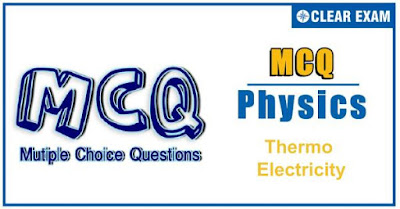##Thermo electricity- MCQ-Basic

Physics is an important subject in preparation for various Competitive exams. To keep a track of your preparation we have designed a small quiz of 10 Basic Level Questions on Thermo electricity.You have 10 minutes to solve the quiz, click the start timer button in order to start the timer and then click on submit button at the end to submit the quiz and view your scores and correct answers.

Q1. If the cold junction of thermocouple is lowered, then the neutral temperature

•  Increases
•  Approaches inversion temperature
•  Decreases
•  Remains the same

Remains the same

Q2. A thermoelectric refrigerator works on

•  Joule effect
•  Seebeck effect
•  Peltier effect
•  Thermionic emission

Peltier effect

Q3. The neutral temperature of a thermocouple is 350.C when the cold junction is at 0.C.When the cold junction is immersed in a bath of 30.C ,the inversion temperature is

•   700.C
•   600.C
•   350.C
•   670.C

670.C

Q4. Two ends of a conductor are at different temperatures the electromotive force generated between two ends is

•  Seebeck electro motive force (emf)
•  Peltier electro motive force (emf)
•  Thomson electro motive force (emf)
•  None of these

Thomson electro motive force (emf)

Q5. For a thermocouple the neutral temperature is 270o C when its cold junction is at 20o C. What will be the neutral temperature and the temperature of inversion when the temperature of cold junction is increased to 40o C

•  290oC , 580oC
•  270oC , 580oC
•  270oC , 500oC
•  290oC , 540oC

270oC , 500oC

Q6. In a Cu-Fe thermo couple the battery current is driven from Cu-Fe through J2. Then

•  J2 should heat’s up
•  J2 should cool down
•  J1 should cool down
•  Both J1 and J2 either heat’s up or cool down depending upon the direction of current

J2 should cool down

Q7. For a thermocouple if the cold junction is maintained at 0oC the inversion temperature is 680oC. Its Neutral temperature is

•  1360oC
•  650oC
•  340oC
•  170oC

340oC

Q8. Consider the following two statements A and B, and identify the correct choice of given answers. A. Thermo e.m.f. is minimum at neutral temperature of a thermocouple B. When two junctions made of two different metallic wires are maintained at different temperatures, an electric current is generated in the circuit.

•  A is false and B is true
•  A is true and B is false
•  Both A and B are false
•  Both A and B are true

A is false and B is true

Q9. In the Seebeck series Bi occurs first followed by Cu and Fe among other. The Sb is the last in the series. If E1 be the thermo emf at the given temperature difference for Bi–Sb thermocouple and E2 be that for Cu–Fe thermocouple, which of the following is true

•  E1 = E2
•  E1 < E2
•  E1 > E2
•  Data is not sufficient to predict it

E1 > E2

Q10. A thermo couple of resistance 2.6 Ω is in series with a meter of resistance 7.4 Ω. It can produce 10 uV/oC difference between junctions. The meter reads 10 mV when a junction is at 0oC and the other junction is in molten metal. Temperature of molten metal is

•  1350oC
•  1500oC
•  1000oC
•  1850oC

1350oC## Want to know more

Want to Know More
Please fill in the details below:

## Latest NEET Articles\$type=three\$c=3\$author=hide\$comment=hide\$rm=hide\$date=hide\$snippet=hide

Name

ltr
item
Best IIT JEE Coaching Institute in Delhi | NEET Coaching Institute in Delhi: Thermo electricity- MCQ-Basic
Thermo electricity- MCQ-Basic